## Montreal R User Group: ggplot2 & rpivotTable

I recently gave a talk at the Montreal R User Group about my favourite data visualization library, ggplot2, as well as rpivotTable, the R interface to my own PivotTable.js

As you can see in the video above, during the talk I just scrolled through an R file in RStudio. What you see below is the result of slightly modifying that file and running it through the RMarkdown process to capture the output.

### Agenda

• ggplot2
• rpivotTable

• I work at Datacratic: a machine learning startup based here in Montreal
• We built the Machine Learning Database, check it out at http://mldb.ai
• I wrote PivotTable.js which is a Javascript pivot table which you’ll see in a bit

• Do you use R every week? CLI or Rstudio?
• Have you ever used ggplot?
• Kept using it, never used it again, sometimes?
• Have you ever used the Pivot Table in Excel? Some other tool?

### ggplot2

• I’m a dataviz nerd: I try every new library/technique
• I usually work in Python, but I always come back to R
• why? ggplot2, it’s the goldilocks dataviz tool!
• higher-level than a drawing tool
• lower-level than a charting tool

#### does nothing

``library(ggplot2)``
``ggplot(mtcars) ``

#### bar chart

``````ggplot(mtcars) +
geom_bar( aes(x=factor(cyl)) )``````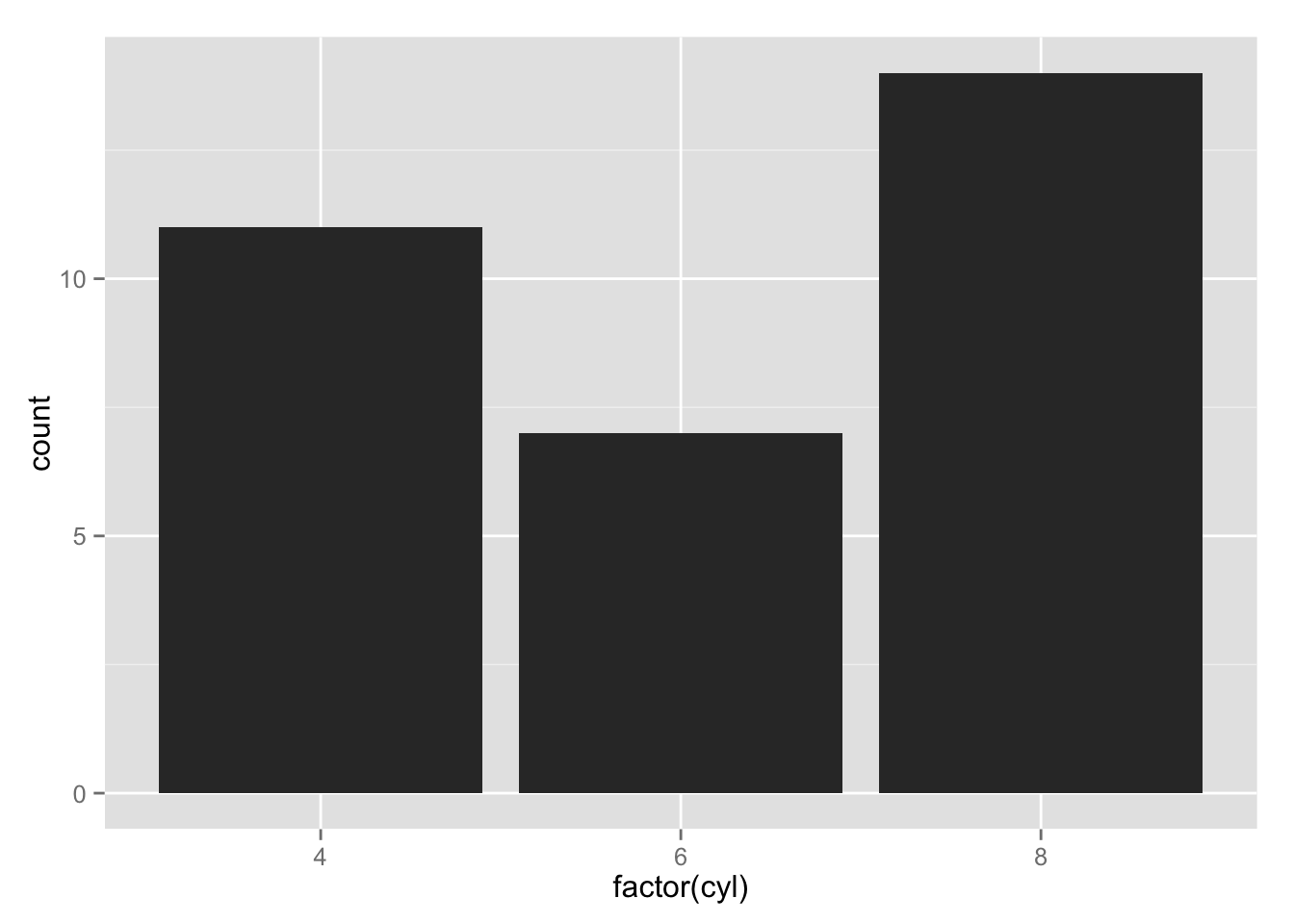#### stacked bar chart

``````ggplot(mtcars) +
geom_bar( aes(x=factor(cyl), fill=factor(gear)) )``````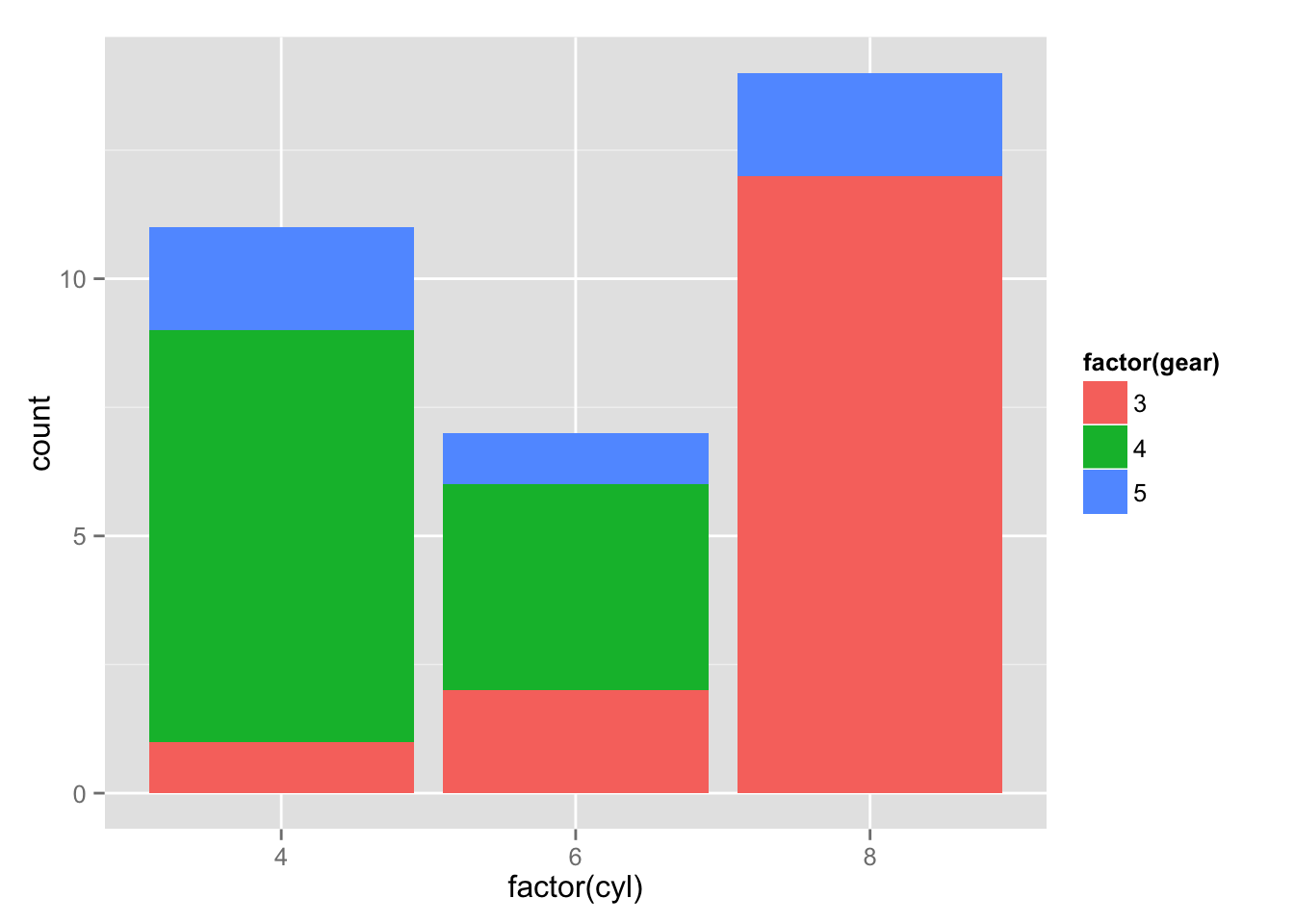#### grouped bar chart

``````ggplot(mtcars) +
geom_bar( aes(x=factor(cyl), fill=factor(gear)), position="dodge" )``````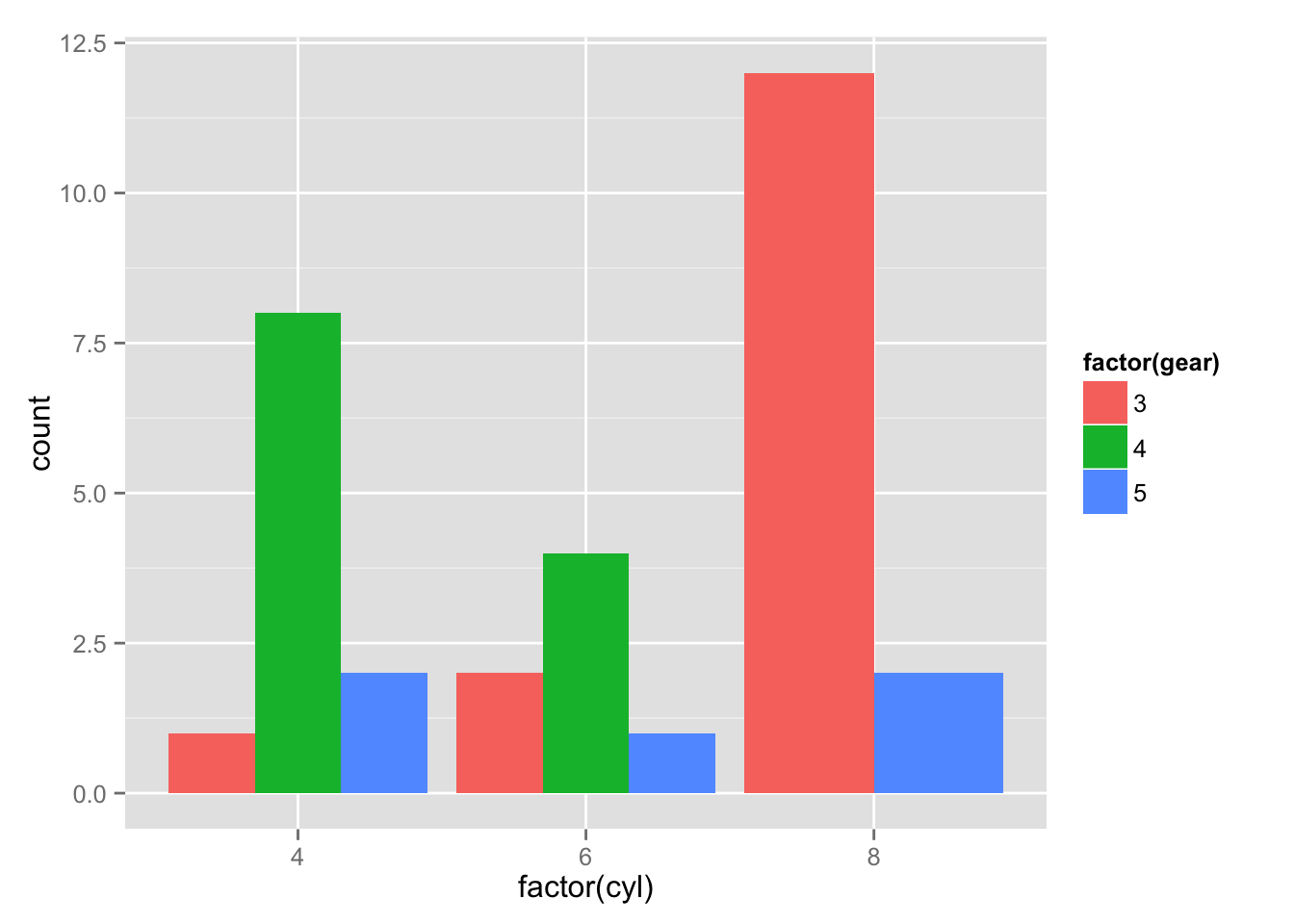#### coloured bar chart

``````ggplot(mtcars) +
geom_bar( aes(x=factor(cyl), fill=factor(cyl)) )``````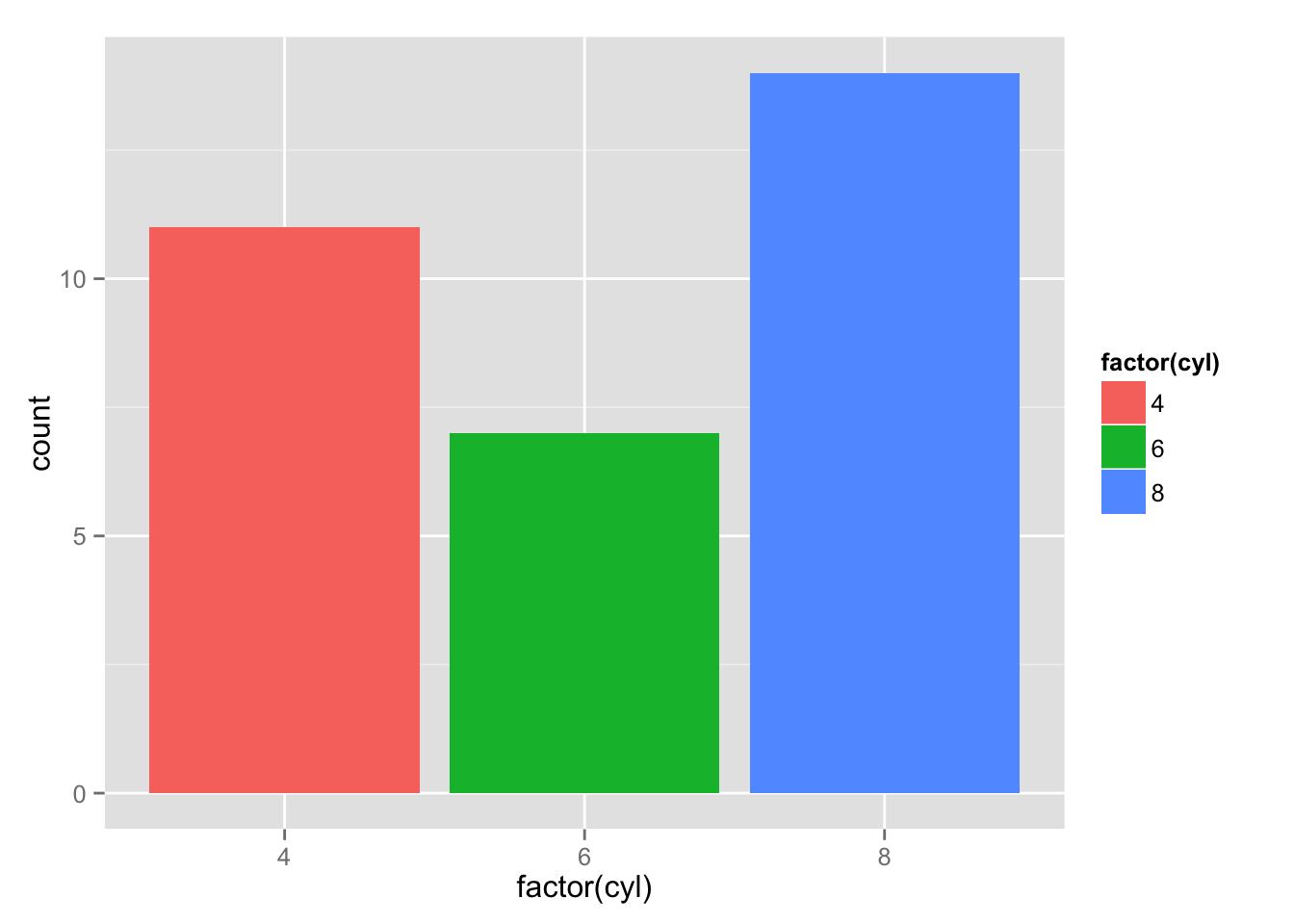#### one big stack, full width…

``````ggplot(mtcars) +
geom_bar( aes(x=factor(1), fill=factor(cyl)), width=1 )``````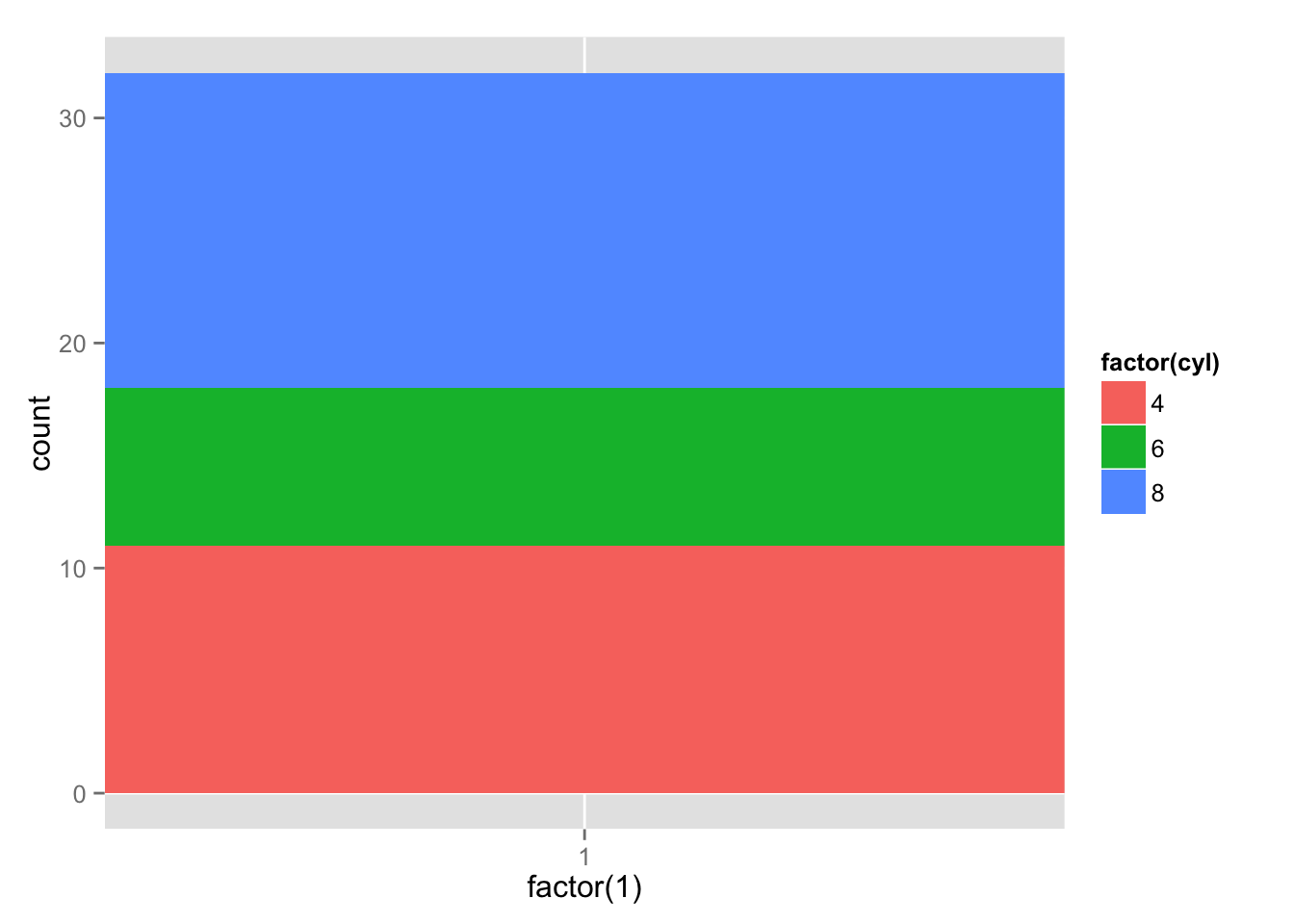#### …in polar coordinates -> pie chart!

``````ggplot(mtcars) +
geom_bar( aes(x=factor(1), fill=factor(cyl)), width=1) +
coord_polar(theta="y")``````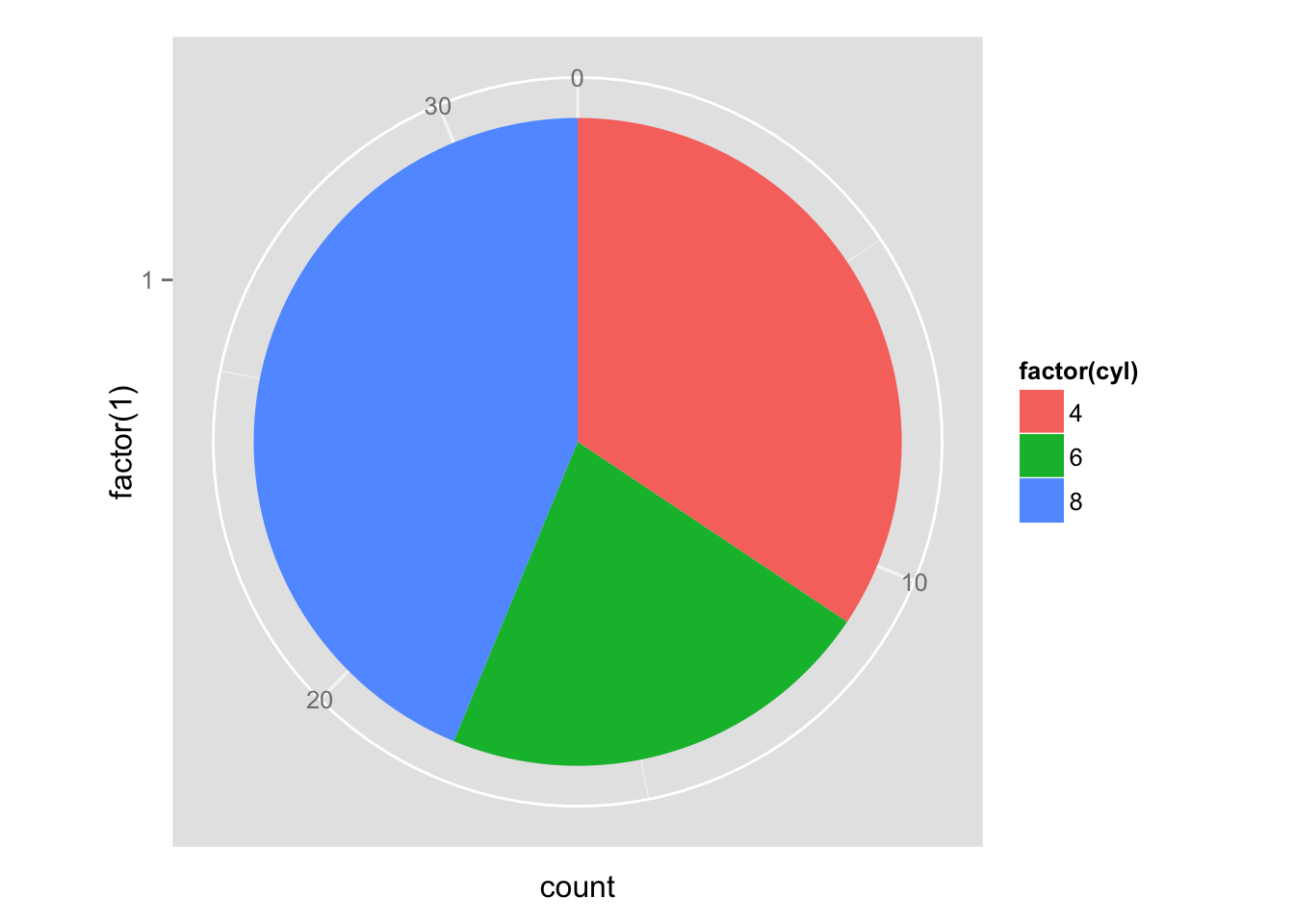#### scatterplot

``````ggplot(mtcars) +
geom_point( aes(x=mpg, y=disp) )``````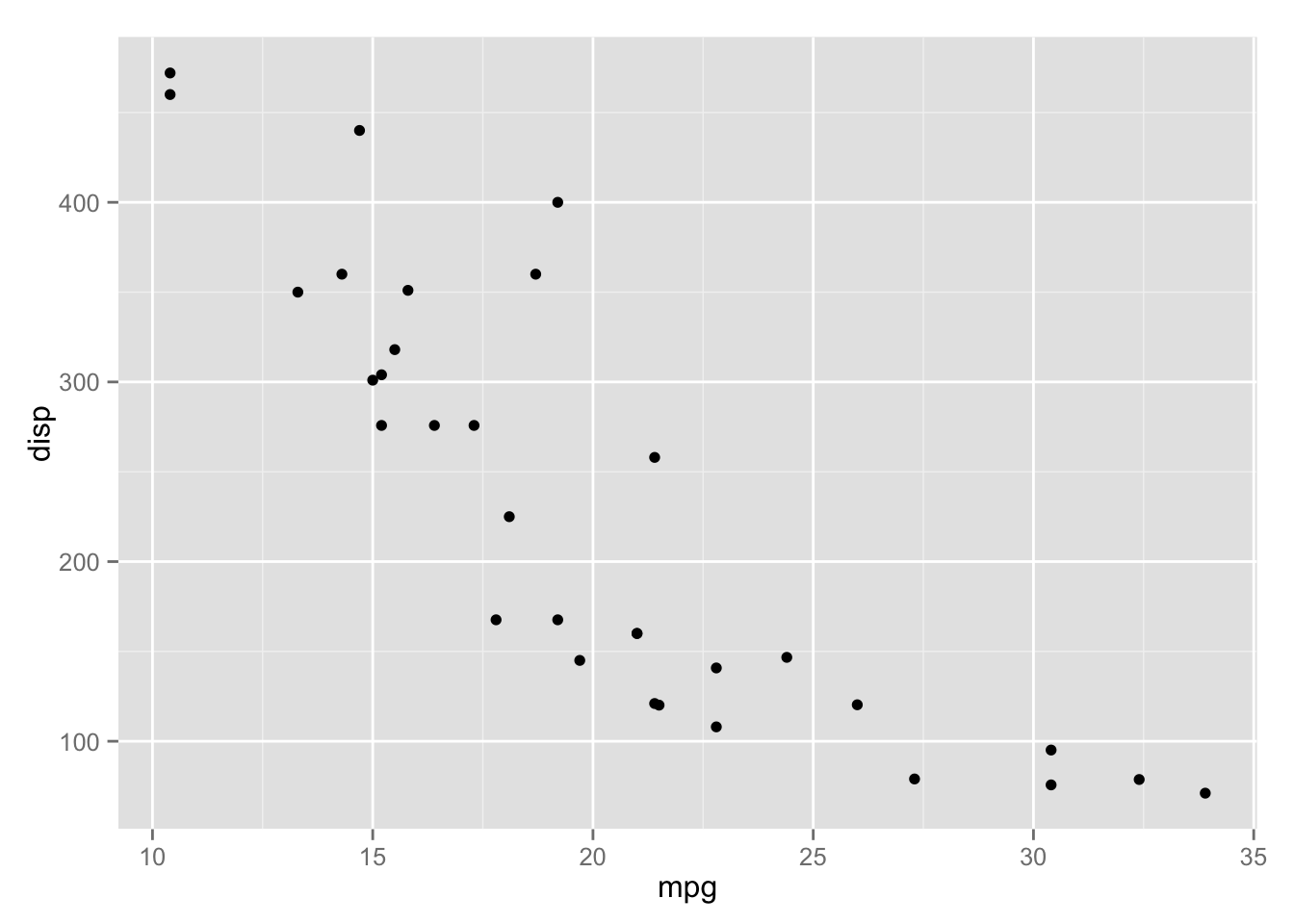#### scatterplot with LOESS

``````ggplot(mtcars) +
geom_point( aes(x=mpg, y=disp) ) +
geom_smooth( aes(x=mpg, y=disp) )``````
``## geom_smooth: method="auto" and size of largest group is <1000, so using loess. Use 'method = x' to change the smoothing method.``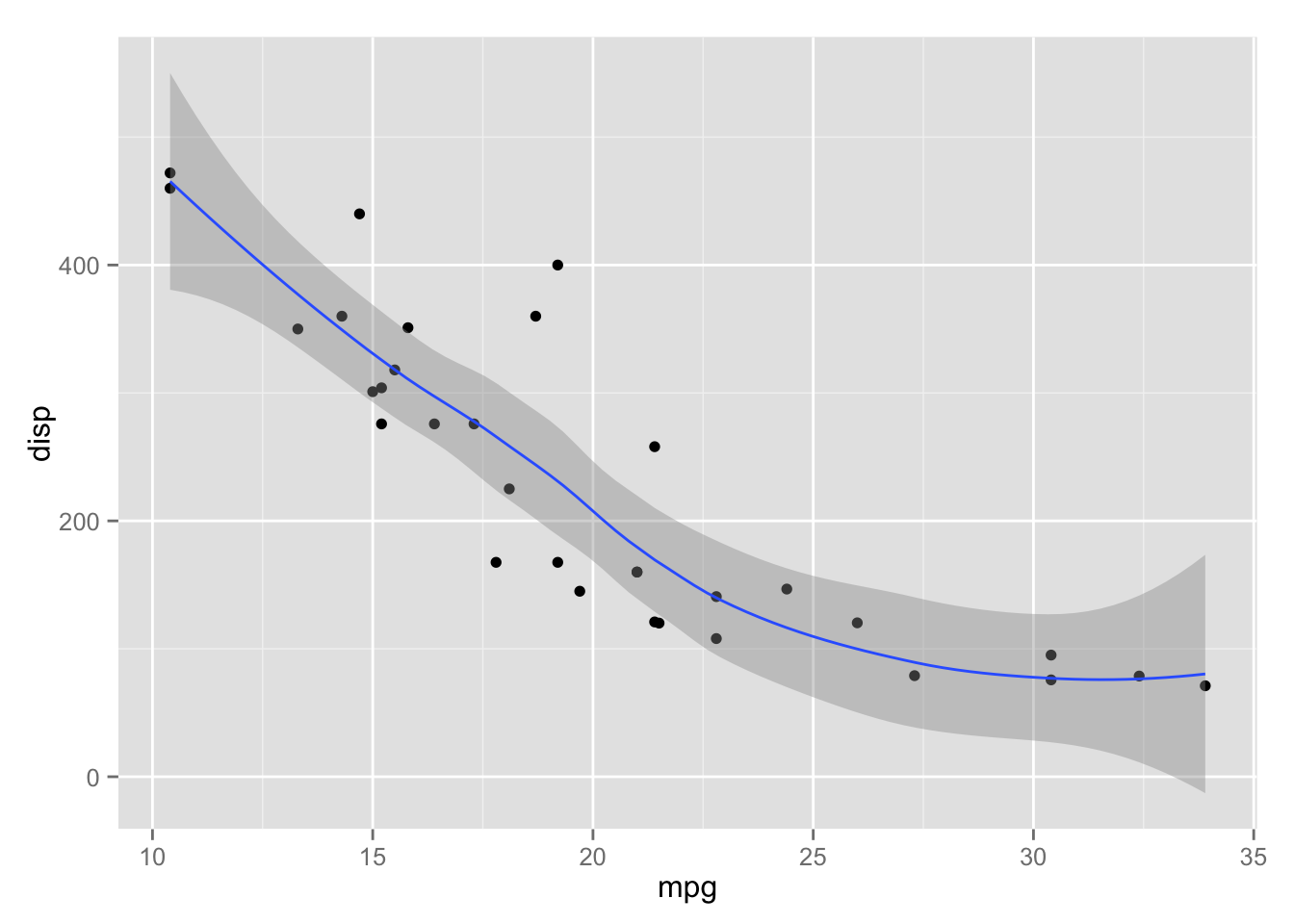#### less repetition

``````ggplot(mtcars, aes(x=mpg, y=disp)) +
geom_point() + geom_smooth()``````
``## geom_smooth: method="auto" and size of largest group is <1000, so using loess. Use 'method = x' to change the smoothing method.``#### colours

``````ggplot(mtcars, aes(x=mpg, y=disp, color=factor(cyl))) +
geom_point() + geom_smooth()``````
``## geom_smooth: method="auto" and size of largest group is <1000, so using loess. Use 'method = x' to change the smoothing method.``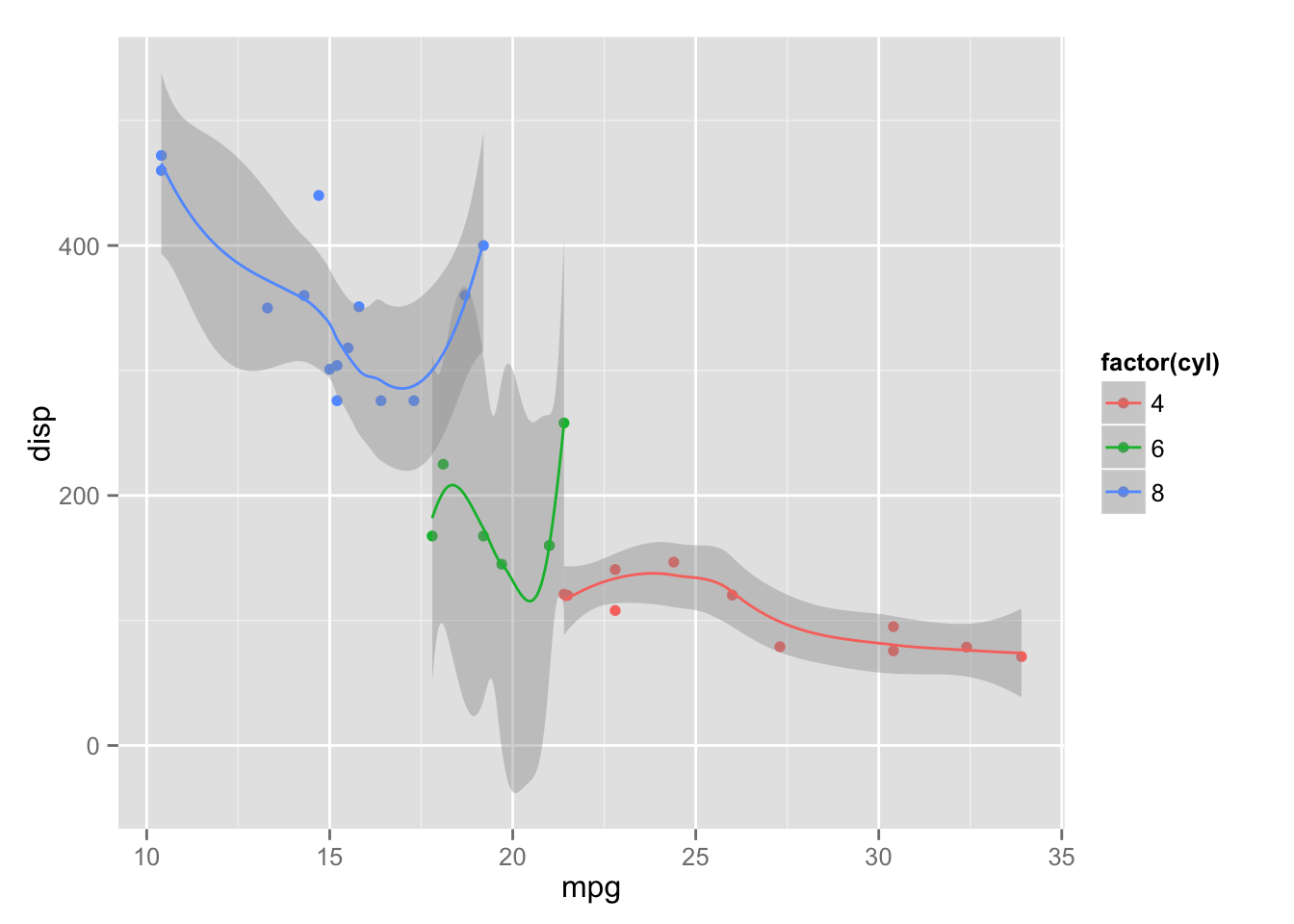#### one big LOESS across colours

``````ggplot(mtcars, aes(x=mpg, y=disp)) +
geom_point( aes(color=factor(cyl)) ) + geom_smooth()``````
``## geom_smooth: method="auto" and size of largest group is <1000, so using loess. Use 'method = x' to change the smoothing method.``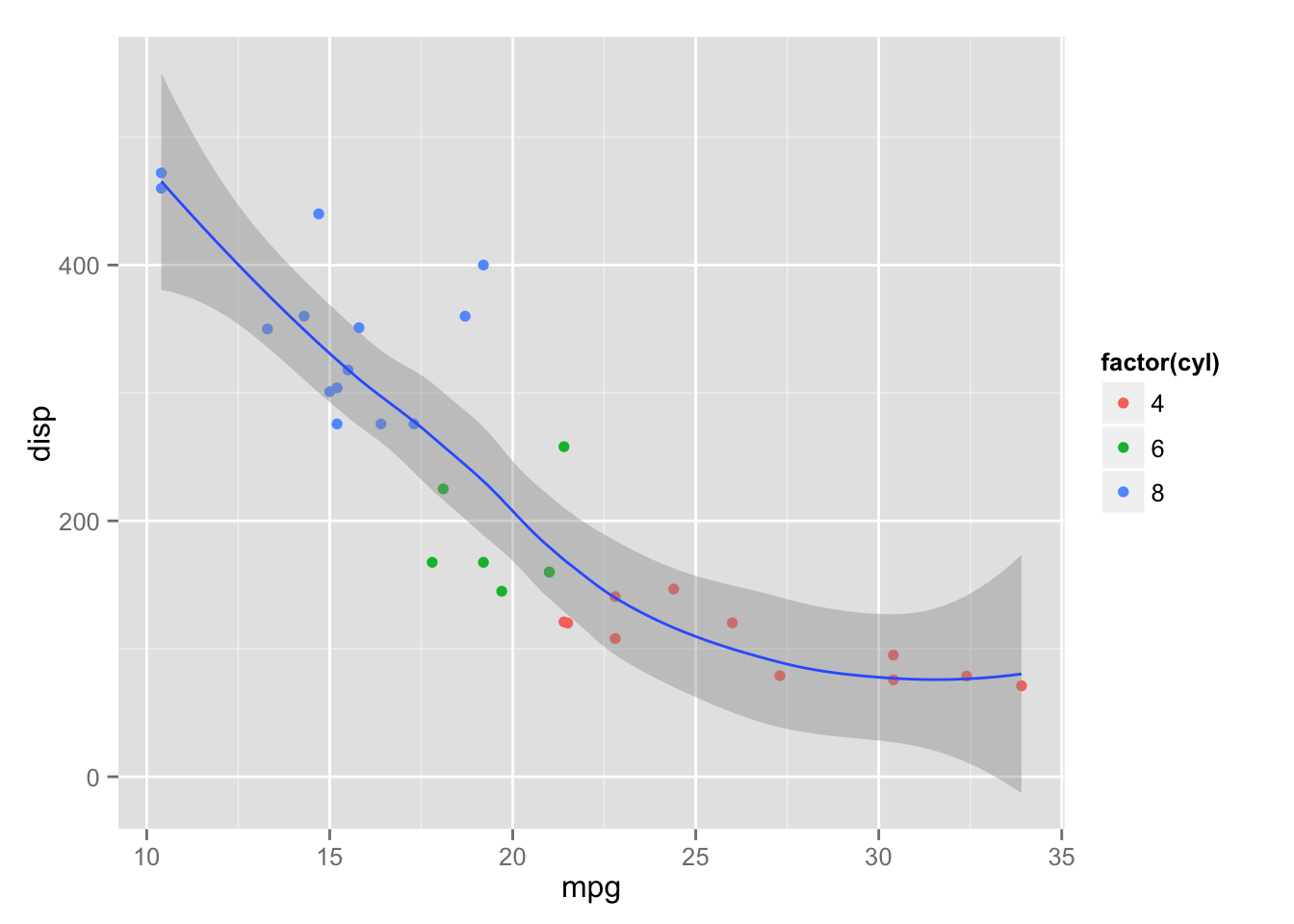#### making the points bigger

``````ggplot(mtcars, aes(x=mpg, y=disp)) +
geom_point( aes(color=factor(cyl)), size=4) + geom_smooth()``````
``## geom_smooth: method="auto" and size of largest group is <1000, so using loess. Use 'method = x' to change the smoothing method.``